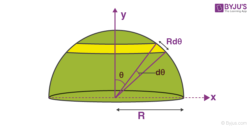Checkout JEE MAINS 2022 Question Paper Analysis : Checkout JEE MAINS 2022 Question Paper Analysis :

# Centre of Mass of Hollow Hemisphere

Centre of the mass of the body is the single point at which the entire mass of the body is imagined to be concentrated and all the forces applied act at that point.

The centre of mass is that point that moves when external forces are applied to the body. The centre of mass will have a translational motion under the influence of forces.

## How to Find Centre of Mass of Hollow HemisphereWe are having a hollow hemisphere of mass M and radius R. The centre of mass of the hollow hemisphere will lie on the y-axis, which is the line passing through the centre of the base of the hollow hemisphere.

We are considering an elemental strip of width Rdθ and has a mass dM. The radius of the elemental ring is r = Rsinθ.

The elemental mass dM = (M/2πR2) (2πRsinθ.Rdθ)

dM = Msinθdθ

The y-coordinate of the centre of mass yc =(1/M)∫ydM

Putting the values of y and dM, we get

yc =(1/M)∫(Rcosθ)(Msinθdθ)

$$\begin{array}{l}y_{c}=\frac{1}{M}\int_{0}^{\pi /2}(Rcos\theta )(Msin\theta d\theta )\end{array}$$

$$\begin{array}{l}y_{c}=\frac{1}{M}\int_{0}^{\pi /2}(Rcos\theta )(Msin\theta d\theta ) \times \frac{2}{2}\end{array}$$

$$\begin{array}{l}y_{c}=\frac{R}{2}\int_{0}^{\pi /2}(sin2\theta d\theta )\end{array}$$

= R/2(½ + ½) = R/2

Therefore, the centre of mass of a hollow hemisphere will be at R/2 along the y-axis. Here R is the radius of the hollow hemisphere.

Test Your Knowledge On Centre Of Mass Of Hollow Hemisphere!